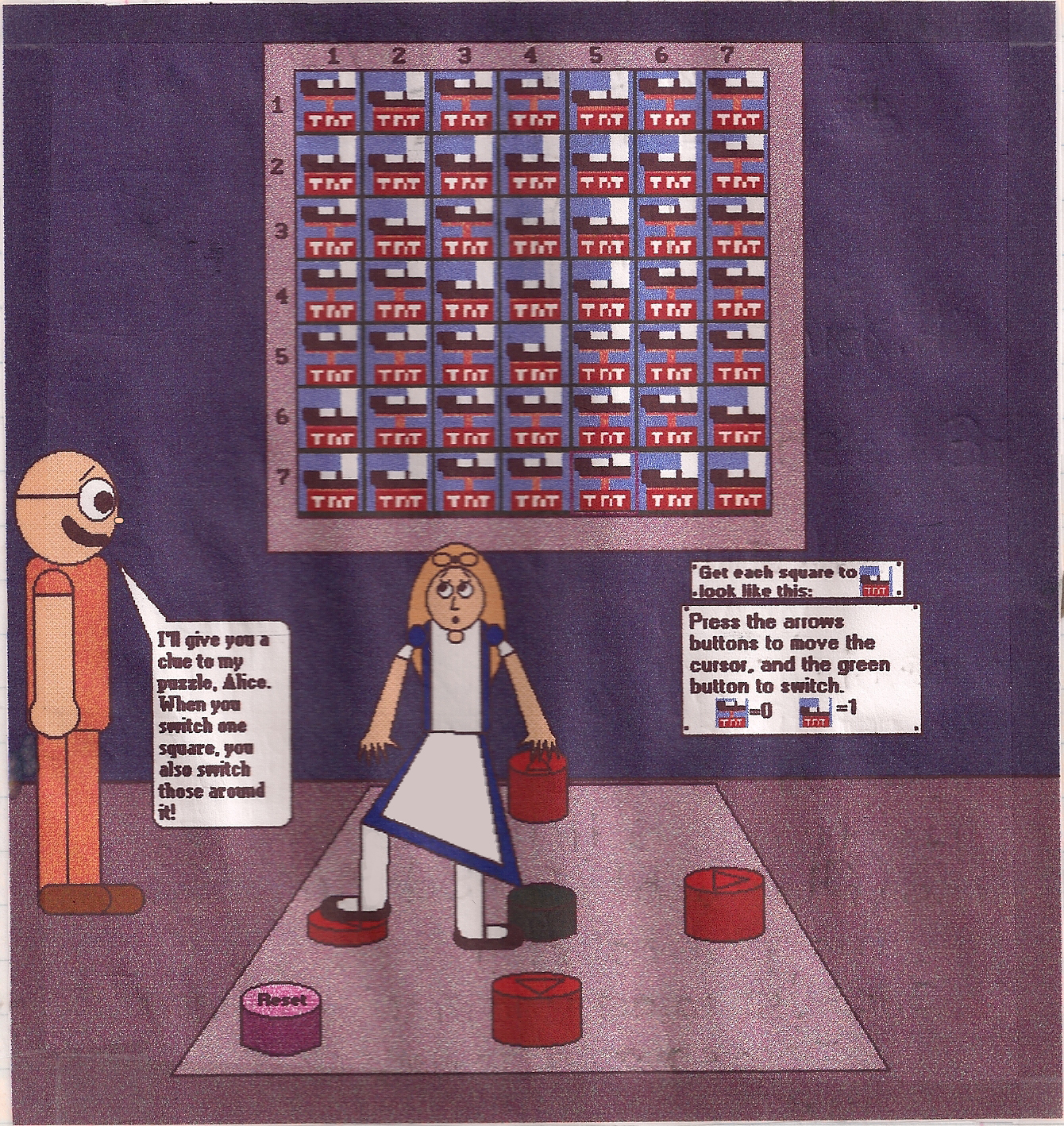# Dr. Foolish & Alice Liddell's Binary Puzzle

#### Difficulty: MediumAlice is trying to solve Dr. John Von Foolish's binary puzzle! She's playing a game in which she has to turn all of those switches on! Dr. Foolish explains how in the image. However, your mission is to calculate the binary value of each row in the picture. If you've learned how to do binary, then you should understand this puzzle, but the clues below will give you an idea, just in case.

### Clues:

1. One clue is in the picture!
2. (64a + 32b + 16c + 8d + 4e + 2f + g) = Binary value of row.
3. a = 1st square of row, b = 2nd square of row, c = 3rd square of row, d = 4th square of row, e = 5th square of row, f = 6th square of row, g = 7th square of row.

### Questions:

1. What's the binary value of Row #1?
2. What's the binary value of Row #2?
3. What's the binary value of Row #3?
4. What's the binary value of Row #4?
5. What's the binary value of Row #5?
6. What's the binary value of Row #6?
7. What's the binary value of Row #7?